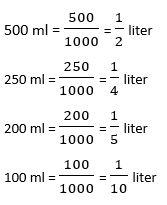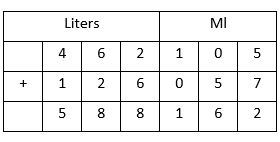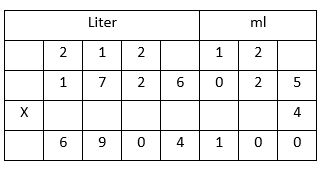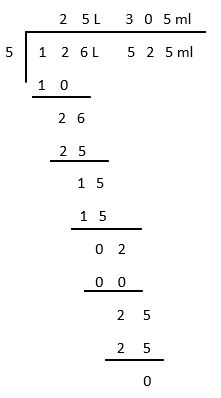## LetsPlayMaths.Com

WELCOME TO THE WORLD OF MATHEMATICS

# Class 4 Capacity / Volume

Measurement of Capacity

Subtraction if Capacity Measures

Multiplication of Capacity Measures

Division of Capacity Measures

Capacity / Volume Test

Capacity Worksheet

## Measurement of Capacity

The standard unit for measuring capacity is liter. Small quantities are measured in milliliters. Liters are written
as ‘L’ and milliliters are written as ‘ml’.
From class III we know that, 1000 milliliters = 1 LiterWe have learnt the addition of capacity measures in class III. Here, we will use the same methods to add the
bigger quantities. We can add liters and milliliters like normal numbers, but milliliters should be written as
three digits numbers. For example, 45 milliliters should be written as 045 milliliters and 5 milliliters should
be written as 005 milliliters.

Let’s have a look at some examples.

Example 1. Add 462 L 105 ml and 126 L 57 ml.
Solution.So, the answer is 588 L 162 ml.

Example 2. Add 1471 L 750 ml, 302 L 250 ml and 76 L 90 ml.
Solution.So, the answer is 1850 L 90 ml.

## Subtraction if Capacity Measures

We can subtract liters and milliliters as normal subtraction. But, milliliters should be written as three-digit numbers.
Let’s have a look at some examples.

Example 1. Subtract 1562 L 96 ml from 2162 L 746 ml.
Solution. Write 2162 L 746 ml and 1562 L 96 ml in tabular format.So, the answer is 600 L 650 ml.

## Multiplication of Capacity Measures

We can multiply liters and milliliters as normal multiplication. But, milliliters should be written as three-digit numbers.
Let’s have a look at some examples.

Example 1. Multiply 1726 L 25 ml by 4.
Solution. Write 1726 L 25 ml and 3 in tabular format as shown below.So, the answer is 6904 L 100 ml.

## Division of Capacity Measures

Division of liters and milliliters can be done with normal division. In this method, milliliters must be written in 3-digit format.

Example 1. Divide 126 L 525 ml by 5.
Solution.So, the answer is 25 L 305 ml.

## Capacity / Volume Test

Capacity / Volume Test - 1

Capacity / Volume Test - 2

## Class-4 Capacity/Volume Worksheet

Capacity Worksheet - 1

Capacity Worksheet - 2

Capacity Worksheet - 3